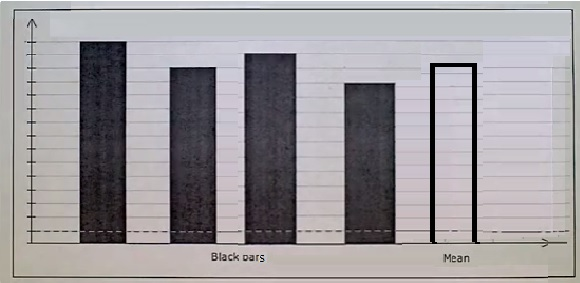# Understanding the Mean Graphically: Four or more bars

In this lesson we understand the mean of a dataset using graphical method. Suppose we are given a bar graph showing four bars of data. Here we are required to find the mean of given data graphically.

## Rules to find the mean graphically

• In the bar graph we find the heights of the four bars.

• The average or mean of these heights is found.

• We then draw a fifth bar with the average height found in second step.

• The height of this fifth bar gives the mean or average of given data set graphically.The four bars in a bar graph have heights 14, 16, 18, and 22. What height a new bar should have so that it is the mean height of the four bars?

### Solution

Step 1:

Heights of given bars 14, 16, 18 and 22

Step 2:

Mean height = $\frac{(14 + 16 + 18 + 22)}{4} = \frac{70}{4}$ = 17.5

So height of new bar = 17.5.

The four bars in a bar graph have heights 15, 16, 17 and 20. What height a new bar should have so that it is the mean height of the four bars?

### Solution

Step 1:

Heights of given bars 15, 16, 17 and 20

Step 2:

Mean height = $\frac{(15 + 16 + 17 + 20)}{4} = \frac{68}{4}$ = 17

So height of new bar = 17